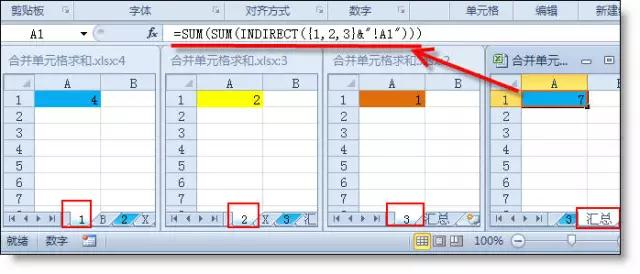#### 用Sum函数的小伙们请看过来!

1、自动批量设置Sum求和公式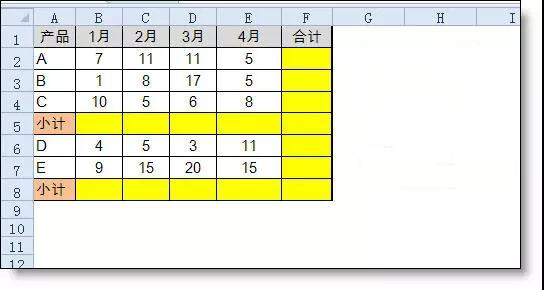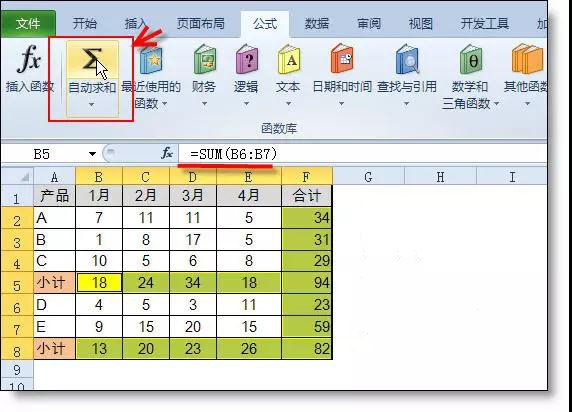2、巧设置总计公式。

=SUM(B2:B14)/2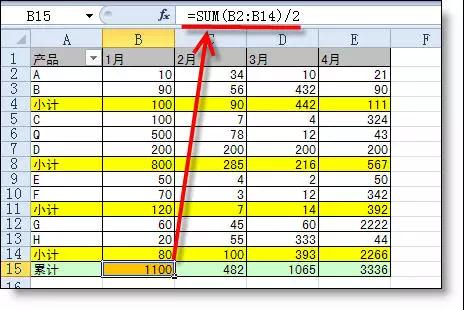3、多表求和

=Sum(1日:31日!B2)

4、多条件求和

{=Sum((a1:a100="A产品")*b1:b100)}

5、合并单元格求和

=SUM(C2:C13)-SUM(D3:D13)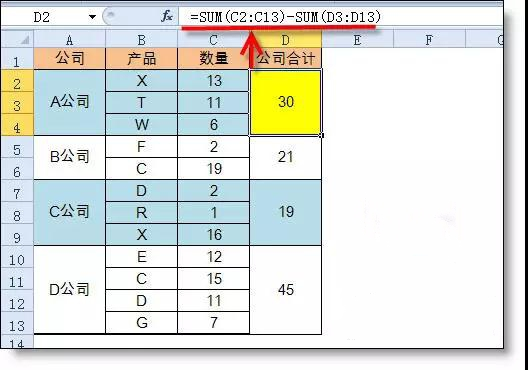6、任意多表求和

=SUM(SUM(INDIRECT({1,2,3}&"!A1")))

=SUM(N(INDIRECT({1,2,3}&"!A1")))